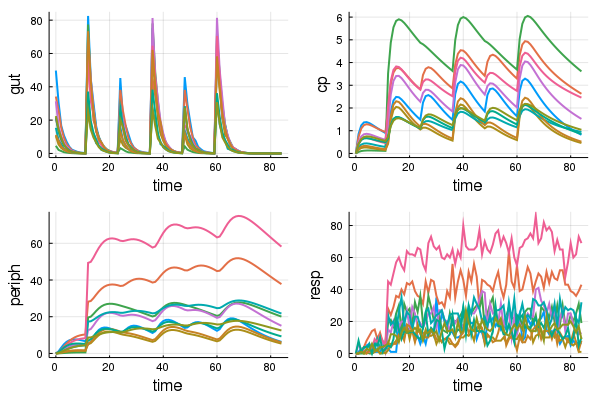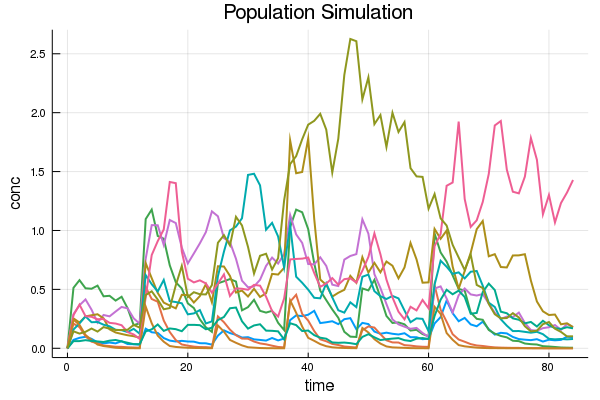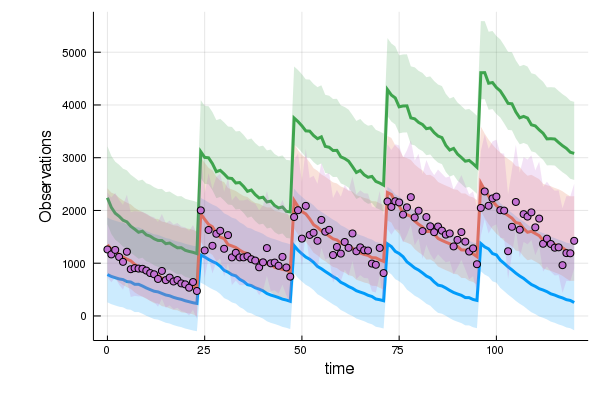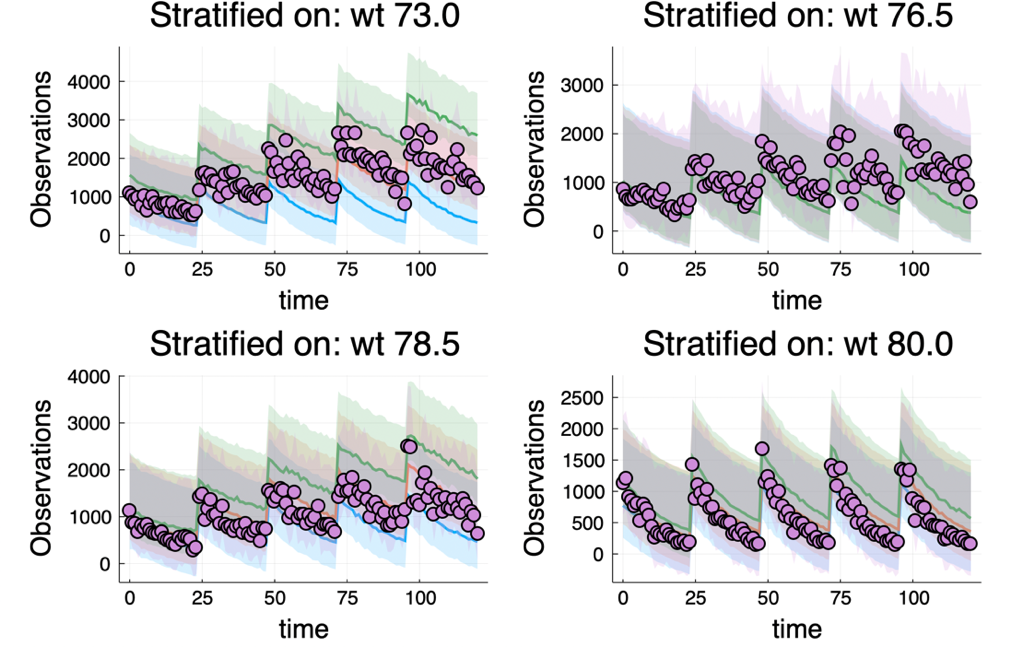#### Introducing Pharmaceutical Modeling & Simulation with Pumas

19 Jul 2019 | Chris Rackauckas, Vijay Ivaturi, Joga Gobburu

##### …Then we found you a solution!

Pumas is a new tool for Pharmaceutical Modeling and Simulation which allows for integrating the workflows across the spectrum of pre-clinical and clinical analysis. The modules of Pumas are all designed to work together, allowing for the Noncompartmental Analysis (NCA) module to be directly used in Nonlinear Mixed Effects (NLME) simulations and estimations, be piped directly to Bioequivalence analysis, or be a central measure in In-Vitro In-Vivo Correlation (IVIVC). Not only does this compatibility allow the use of a single software to be used throughout the quantitative workflow, but it also allows for new workflows to be explored, such as Bayesian NLME on quantitative systems pharmacology (QsP) models and global sensitivity analysis of NCA-derived quantities.

None of this would be usable without the performance to handle complex real-world models and data. This is where Pumas shines: building off of the battle-tested DifferentialEquations.jl library to deliver a wide array of robust and performance-optimized differential equation solving, along with the differentiable programming and JIT compilation of the Julia language, to deliver outcomes that routinely outperform the classic software of the respective domains. Together with the internal parallelism and GPU-compatibility of the methods, Pumas hopes to help the pharmacometric community transition to a world of increasingly complex models and even bigger data.

In this blog post we will describe the reasons Pumas was created, and the features that have been developed. These features will be released on a staggered schedule to start gathering user feedback and allow for rapid development. We stress that this is the alpha release of Pumas, and we welcome users to give it a try!

##### Why Julia?

Pumas is built using the Julia programming language. This was not an arbitrary choice and is instead one of the fundamental reasons Pumas was able to be created. In the field of pharmacometrics and other releated quantitative sciences used in drug devleopment, we see the need for our programming interface to be interactive, allowing users to explore models and data in an intuitive manner. However, most dynamic programming languages, such as R and Python, have significant performance disadvantages compared to compiled languages like C++ and Fortran, a fact which has splintered the workflows of pharmacometric models. Nevertheless, Julia stands in the middle as an interactive language which is able to achieve the speeds of Fortran, allowing it to fill this much needed gap for the community.

There are many additional reasons why Julia has been found to be favorable. While most of the differential equation solver libraries that are central to pharmacometric models were developed and untouched since the 70’s and 80’s, the Julia community is unique in that it has a thriving community of open source differential equation methods and software developers in the JuliaDiffEq organization. The culminating software, DifferentialEquations.jl, has been shown to have many unique and advantageous algorithms for handling stiff hybrid differential equation systems which arise in pharmacokinetic/pharmacodynamic (PK/PD), physiologically-based pharmacokinetic (PBPK), and QsP models. This software ecosystem is undergoing continual advances in the areas of parallelism (multithreaded, distributed, and GPU), improved solver strategies like IMEX and exponential integrators, along with advancing the methodologies in new mathematical modeling areas like delay differential equations (DDEs), stochastic differential equations (SDEs), and jump equations (Gillespie).

Coupling this software with the differentiable programming and optimization libraries of the Julia language allows for Pumas to be a 1-language solution which not only offers a wide feature set but also deliver high performance and parallelism.

Let’s take a deep dive into nonlinear mixed effects models with Pumas and show how these integrate with various other tools to give a one-software solution to pharmacometric analysis workflows.

##### Installation is a Breeze!

We do not mess around with compilers, build processes, making sure multiple languages can communicate, etc. Because Pumas is written entirely in Julia, our modeling suite is as simple to install and run as any other Julia code. Pumas can be acquired through JuliaPro, where once the binary auto-install is complete, you run the command:

``````using Pkg
``````

and now Pumas is installed. This works on Windows, Mac, and Linux and on all CPU architectures that Julia is compatible with!

##### Simulating NLME Models with Pumas

The core of Pumas is the ability to build nonlinear mixed effects models. For details, please see the first tutorial of the documentation. These models are represented in a simplified form by the `@model` macro. A multiple-response PK/PD model can be defined using this format as follows:

``````using Pumas, LinearAlgebra
f(x,y) = x*exp(y)

mymodel = @model begin
@param   θ ∈ VectorDomain(12)
@random  η ~ MvNormal(Matrix{Float64}(0.1I, 11, 11))

@pre begin
Ka      = θ
CL      = θ*exp(η)
Vc      = f(θ,η)
Q       = θ*exp(η)
Vp      = θ*exp(η)
Kin     = θ*exp(η)
Kout    = θ*exp(η)
IC50    = θ*exp(η)
IMAX    = θ*exp(η)
γ       = θ*exp(η)
Vmax    = θ*exp(η)
Km      = θ*exp(η)
end

@init begin
Resp = Kin/Kout
end

@dynamics begin
Gut'    = -Ka*Gut
Cent'   =  Ka*Gut - (CL+Vmax/(Km+(Cent/Vc))+Q)*(Cent/Vc)  + Q*(Periph/Vp)
Periph' =  Q*(Cent/Vc)  - Q*(Periph/Vp)
Resp'   =  Kin*(1-(IMAX*(Cent/Vc)^γ/(IC50^γ+(Cent/Vc)^γ)))  - Kout*Resp
end

@derived begin
gut    = @. Normal(Gut,Gut/10)
cp     = Cent / θ
periph = Periph
resp   = @. Poisson(Periph)
end
end
``````

This example highlights some of the many interesting new features provided by Pumas. Unlike many other pharmacometric modeling suites, this macro is building code that is executed from within the same language, meaning that it is compatible with standard Julia constructs, including variables and functions that you the user have defined such as `f`. In addition, any distribution can be given for the error function, making it easy to switch between continuous and discrete observables. For a more detailed description of the `@model` macro, please see the documentation.

Once a model has been defined, one can build a population by either reading in data via the `read_pumas` function (compatible with NMTRAN inputs), or by generating datasets. Let’s showcase the latter. The `DosageRegimen` constructor allows a user to generate regimens directly from Julia code. For example, a population to simulate with differing covariates and randomized steady-state dosing can be generated via:

``````pop = Population([Subject(id = id,
evs = DosageRegimen([50rand(), 100rand()],
ii = 24, addl = 2, ss = 0:1, time = [0, 12],
cmt = 1),cvs = cvs=(isPM="no", Wt=rand()*70)) for id in 1:10])
``````

Now, one can simulate our model by passing parameter values into `simobs`. For example:

``````param = (θ = [
0.5, # Ka  Absorption rate constant 1 (1/time)
1, # CL   Clearance (volume/time)
20, # Vc   Central volume (volume)
2, # Q    Inter-compartmental clearance (volume/time)
10, # Vp   Peripheral volume of distribution (volume)
10, # Kin  Response in rate constant (1/time)
2, # Kout Response out rate constant (1/time)
2, # IC50 Concentration for 50% of max inhibition (mass/volume)
1, # IMAX Maximum inhibition
1, # γ    Emax model sigmoidicity
0, # Vmax Maximum reaction velocity (mass/time)
2  # Km   Michaelis constant (mass/volume)
],)
obs = simobs(mymodel, pop, param)
``````

The results of all Pumas operations includes a 1-line plotting command `plot`. For example, here `plot` shows us the simulated observations:

``````using Plots
plot(obs)
``````The `plot` command is fully tweakable and universal: use it on any Pumas returned type and get the appropriate plot for that domain.

Under the hood, `simobs` is using DifferentialEquations.jl and allows the user to use any of the differential equation solver’s options. Thus for example, we can lower the tolerances and solve using a semi-implicit method for stiff ODEs via:

``````obs = simobs(mymodel, subject, param, abstol = 1e-8, reltol = 1e-8, alg = Rodas5())
``````

One can change parameters on the fly to understand the behavior under different domains. For example, to simulate with all random effects zeroed, we can directly pass the values:

``````randeffs = (η = zeros(11),)
obs = simobs(mymodel, pop, param, randeffs)
``````
##### A Focus on Performance: Efficiency and Scalable Parallelism

All of the power of DifferentialEquations.jl is thus directly embedded into Pumas, including its automatic stiffness detection methods (the default), its Jacobian reuse techniques, and its ModelingToolkit.jl symbolic framework for automated performance enhancements. This function also automatically utilizes Julia’s multithreading, parallelizing across the cores of your computer.

One can additionally setup Pumas to run parallelized over the thousands of cores of a supercomputer. This just utilizes Julia’s built in parallelism support

``````addprocs()
obs = simobs(mmymodel, subject, param, abstol = 1e-8, reltol = 1e-8,
alg = Rodas5(), parallel_type = Pumas.Distributed)
``````

For enterprise user support, Julia Computing offers JuliaTeam+Deployment to help with the deployment and maintenance of large parallel calculations.

Future posts will detail how additional features of DifferentialEquations.jl, such as GPU support, are integrated and exposed for Pumas users.

##### Integrated Noncompartmental Analysis (NCA)

Pumas includes a NCA module which is being battle tested against industry standard tooling. This NCA module is pure Julia which enables it to be fast and allows for specification of units. It directly integrates with the Pumas NLME tooling, allowing for derived variables to be specified as:

``````@derived begin
cp = @. 1000*(Central / V)
nca := @nca cp
auc =  NCA.auc(nca)
thalf =  NCA.thalf(nca)
cmax = NCA.cmax(nca)
end
``````

and thus directly make use of the NCA tooling.

##### Catering to Power Users: Function-Based Interfaces and SDEs/DDEs

The NLME simulation tooling is written entirely in Julia and does not have to be accessed through the indirection of a domain-specific language. Pumas comes will a full-fledged function-based interface which caters to power users interested in controlling every performance detail.

We can then develop a stochastic differential equation model via:

``````using StochasticDiffEq
p = ParamSet((θ=VectorDomain(3, lower=zeros(3)), Ω=PSDDomain(2)))
function randomfx(p)
ParamSet((η=MvNormal(p.Ω),))
end

function pre_f(params, randoms, subject)
θ = params.θ
η = randoms.η
(Ka = θ,
CL = θ*exp(η),
V  = θ*exp(η))
end

function f(du,u,p,t)
Depot,Central = u
du = -p.Ka*Depot
du =  p.Ka*Depot - (p.CL/p.V)*Central
end

function g(du,u,p,t)
du = 0.2u
du = 0.2u
end

prob = SDEProblem(f,g,nothing,nothing)

init_f = (col,t) -> [0.0,0.0]

function derived_f(col,sol,obstimes,subject)
central = sol(obstimes;idxs=2)
conc = @. central / col.V
(conc=conc,)
end

stoch_model = Pumas.PumasModel(p,randomfx,pre_f,init_f,prob,derived_f)
``````

and then simulate the SDE with a high strong order adaptive time stepping SDE solver via:

``````pop = Population([Subject(evs = DosageRegimen([10, 20], ii = 24, addl = 2, time = [0, 12])) for i in 1:10])
param = (θ = [
1.5,  #Ka
1.0,  #CL
30.0 #V
],
Ω = [1.0 0.0; 0.0 1.0])
obs = simobs(stoch_model, pop, param, alg=SOSRI())
``````Similarly, state-dependent delay differential equations (with and without steady state dosing) can be built similarly, and are described in the tutorials. While these models have long been the subject of research, showcasing how they can improve the predictability and reliability of NLME models,

Pumas is the first pharmacometrics library to have full support with stiff adaptive time stepping algorithms and steady state dosing readily available for both stochastic and delay differential equations, and we are interested to see the new research that is possible with this tooling. In fact, Julia is the only language with many of the integration techniques which are utilized to allow Pumas to excel with these modern models.

##### Maximum Likelihood and Bayesian Parameter Estimation for NLME Models

With the ability to simulate NLME models comes the ability to estimate the parameters. Maximum likelihood estimation with the usual approximations:

• FO
• FOCE(I)
• Laplace(I)

are all included with Pumas, using the same modeling interface as simulation. To fit data instead of simulating, one simply changes to using the `fit` function. For example, let’s fit the one-compartment model defined in the Pumas README. We can run the fitting routine against some data:

``````julia> res = fit(model,data,initial_parameters,Pumas.FOCEI())
FittedPumasModel

Successful minimization:                true

Likelihood approximation:        Pumas.FOCEI
Objective function value:            8516.61
Total number of observation records:    1210
Number of active observation records:   1210
Number of subjects:                       10

-----------------
Estimate
-----------------
tvcl    4.4531
tvv    77.547
pmoncl -0.73493
Ω₁,₁    0.037694
Ω₂,₂    0.11752
σ_prop  0.042341
-----------------
``````

Pumas includes all of the inference techniques necessary for analyzing the fit. For example, we can infer the confidence intervals of our fit with the inspect function:

``````julia> infer(myfit)
Calculating: variance-covariance matrix. Done.
FittedPumasModelInference

Successful minimization:                true

Likelihood approximation:        Pumas.FOCEI
Objective function value:            8516.61
Total number of observation records:    1210
Number of active observation records:   1210
Number of subjects:                       10

---------------------------------------------------
Estimate       RSE           95.0% C.I.
---------------------------------------------------
tvcl    4.4531      9.0087 [ 3.6668   ;  5.2394  ]
tvv    77.547      10.988  [60.846    ; 94.247   ]
pmoncl -0.73493    -4.5534 [-0.80052  ; -0.66934 ]
Ω₁,₁    0.037694   31.359  [ 0.014526 ;  0.060862]
Ω₂,₂    0.11752    52.745  [-0.0039693;  0.23901 ]
σ_prop  0.042341    2.6616 [ 0.040132 ;  0.044549]
---------------------------------------------------
``````

Model diagnostics and residuals can then be computed with a one line call to `inspect`:

``````resout = DataFrame(inspect(res))
``````
``````julia> first(resout, 6)
6×13 DataFrame
│ Row │ id     │ time    │ isPM  │ wt    │ pred    │ ipred   │ pred_approx │ wres      │ iwres    │ wres_approx │ ebe_1     │ ebe_2     │ ebes_approx │
│     │ String │ Float64 │ Int64 │ Int64 │ Float64 │ Float64 │ Pumas.FOCEI │ Float64   │ Float64  │ Pumas.FOCEI │ Float64   │ Float64   │ Pumas.FOCEI │
├─────┼────────┼─────────┼───────┼───────┼─────────┼─────────┼─────────────┼───────────┼──────────┼─────────────┼───────────┼───────────┼─────────────┤
│ 1   │ 1      │ 0.0     │ 0     │ 68    │ 1326.95 │ 1290.5  │ FOCEI()     │ 0.0141867 │ 0.164838 │ FOCEI()     │ -0.273173 │ 0.0282462 │ FOCEI()     │
│ 2   │ 1      │ 1.0     │ 0     │ 68    │ 1255.42 │ 1236.44 │ FOCEI()     │ 0.247655  │ 0.414528 │ FOCEI()     │ -0.273173 │ 0.0282462 │ FOCEI()     │
│ 3   │ 1      │ 2.0     │ 0     │ 68    │ 1187.56 │ 1184.65 │ FOCEI()     │ -1.44113  │ -1.53356 │ FOCEI()     │ -0.273173 │ 0.0282462 │ FOCEI()     │
│ 4   │ 1      │ 3.0     │ 0     │ 68    │ 1123.17 │ 1135.03 │ FOCEI()     │ -0.66784  │ -1.10145 │ FOCEI()     │ -0.273173 │ 0.0282462 │ FOCEI()     │
│ 5   │ 1      │ 4.0     │ 0     │ 68    │ 1062.1  │ 1087.49 │ FOCEI()     │ -0.67988  │ -1.29264 │ FOCEI()     │ -0.273173 │ 0.0282462 │ FOCEI()     │
│ 6   │ 1      │ 5.0     │ 0     │ 68    │ 1004.17 │ 1041.94 │ FOCEI()     │ 1.14917   │ 0.521982 │ FOCEI()     │ -0.273173 │ 0.0282462 │ FOCEI()     │
``````

To visualize the variability of the fit on the population, one just calls the visual predictive check (`vpc`) function:

``````vpc(res,200) |> plot
````````````vpc(res,200;stratify_on=[:wt]) |> plot
``````##### Bioequivalence
``````using Bioequivalence, CSV
``````

Here is a simple example using data from a cross over study

``````SLF2014 = CSV.read(string(dirname(pathof(Bioequivalence)),
"/../data/2S2P/SLF2014_8.tsv"),
delim = '\t')
``````
``````julia> first(SLF2014, 6)
6×4 DataFrames.DataFrame
│ Row │ id    │ period │ sequence │ AUC     │
│     │ Int64 │ Int64  │ String   │ Float64 │
├─────┼───────┼────────┼──────────┼─────────┤
│ 1   │ 1     │ 1      │ TR       │ 168.407 │
│ 2   │ 1     │ 2      │ TR       │ 210.919 │
│ 3   │ 2     │ 1      │ TR       │ 131.031 │
│ 4   │ 2     │ 2      │ TR       │ 67.4314 │
│ 5   │ 3     │ 1      │ TR       │ 151.737 │
│ 6   │ 3     │ 2      │ TR       │ 85.1296 │
``````

The bioequivalence analysis can be requested through `BioequivalenceStudy`

``````Crossover = BioequivalenceStudy(SLF2014)
``````

The entire summary of the result can be printed out

``````julia> Crossover
Design: RT|TR

Sequences: RT|TR (2)
Periods: 1:2 (2)
Subjects per Sequence: (RT = 288, TR = 429)

Average Bioequivalence
───────────────────────────────────────────────────────────────────────────────
PE        SE       lnLB        lnUB       GMR        LB       UB
───────────────────────────────────────────────────────────────────────────────
T - R  -0.0680309  0.044609  -0.141501  0.00543947  0.934232  0.868054  1.00545
───────────────────────────────────────────────────────────────────────────────
``````

We cover multiple designs and details can be found at the Bioequivalence documentation

Another example is the common two sequence 4 period design (2S4P)

``````PJ43 = CSV.read(string(dirname(pathof(Bioequivalence)),
"/../data/2S4P/PJ2006_4_3.tsv"),
delim = '\t')
``````
``````julia> first(PJ43,6)
6×5 DataFrames.DataFrame
│ Row │ id    │ sequence │ period │ AUC    │ Cmax   │
│     │ Int64 │ String   │ Int64  │ Int64⍰ │ Int64⍰ │
├─────┼───────┼──────────┼────────┼────────┼────────┤
│ 1   │ 1     │ RTTR     │ 1      │ 10671  │ 817    │
│ 2   │ 1     │ RTTR     │ 2      │ 12772  │ 1439   │
│ 3   │ 1     │ RTTR     │ 3      │ 13151  │ 1310   │
│ 4   │ 1     │ RTTR     │ 4      │ 11206  │ 1502   │
│ 5   │ 2     │ TRRT     │ 1      │ 6518   │ 1393   │
│ 6   │ 2     │ TRRT     │ 2      │ 6068   │ 1372   │
``````

One uses the same API to analyse all designs including NTI and HVD. In this case:

``````Inner = BioequivalenceStudy(PJ43)
Design: RTTR|TRRT

Sequences: RTTR|TRRT (2)
Periods: 1:4 (4)
Subjects per Sequence: (RTTR = 8, TRRT = 9)
Regulatory constant for reference-scaled estimates: 0.10
Auxiliary parameter for reference-scaled estimates: 1.11

Average Bioequivalence
PE        SE        lnLB      lnUB     GMR       LB      UB     scLB    scUB Varratio    VarLB  VarUB
T - R  0.0356935 0.0226361 -0.00230475 0.0736918 1.03634 0.997698 1.07647 0.908307 1.10095  1.29984 0.962939 1.7546
``````
##### Conclusion

Pumas is built from the ground up, utilizing the latest advancements in numerical differential equations, differentiable programming, and optimization to give a robust and efficient platform for doing pharmacometric analysis. Here we have described the NLME aspects of Pumas. Follow up posts will showcase how other modules of Pumas, like QsP and PBPK, compose together to give one unified feel for a unified field of methods.